# Attribute Grammars They extend contextfree grammars to give

• Slides: 22Attribute Grammars • They extend context-free grammars to give parameters to non-terminals, have rules to combine attributes • Attributes can have any type, but often they are trees • Example: – context-free grammar rule: A : : = B C – attribute grammar rules: A : : = B C { Plus(\$1, \$2) } or, e. g. A : : = B: x C: y {: RESULT : = new Plus(x. v, y. v) : } Semantic actions indicate how to compute attributes • attributes computed bottom-up, or in more general wayParser Generators: Attribute Grammar -> Parser 1) Embedded: parser combinators (Scala, Haskell) They are code in some (functional) language implicit conversion: string s to skip(s) def ID : Parser = "x" | "y" | "z" concatenation def expr : Parser = factor ~ (( "+" ~ factor | "-" ~ factor ) <- often not really LL(1) but "try one by one", | epsilon)put first non-empty, then epsilon def factor : Parser = term ~ (( "*" ~ term | "/" ~ term ) | epsilon) def term : Parser = ( "(" ~ expr ~ ")" | ID | NUM ) implementation in Scala: use overloading and implicits must 2) Standalone tools: Java. CC, Yacc, ANTLR, CUP – generate code in a conventional programming languages (e. g. Java)Example in CUP - LALR(1) (not LL(1) ) precedence left PLUS, MINUS; precedence left TIMES, DIVIDE, MOD; // priorities disambiguate precedence left UMINUS; expr : : = expr PLUS expr // ambiguous grammar works here | expr MINUS expr | expr TIMES expr | expr DIVIDE expr | expr MOD expr | MINUS expr %prec UMINUS | LPAREN expr RPAREN | NUMBER ;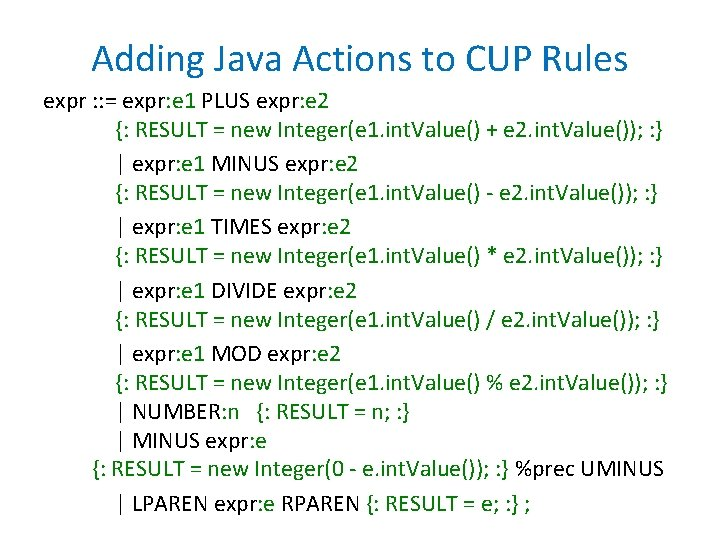Adding Java Actions to CUP Rules expr : : = expr: e 1 PLUS expr: e 2 {: RESULT = new Integer(e 1. int. Value() + e 2. int. Value()); : } | expr: e 1 MINUS expr: e 2 {: RESULT = new Integer(e 1. int. Value() - e 2. int. Value()); : } | expr: e 1 TIMES expr: e 2 {: RESULT = new Integer(e 1. int. Value() * e 2. int. Value()); : } | expr: e 1 DIVIDE expr: e 2 {: RESULT = new Integer(e 1. int. Value() / e 2. int. Value()); : } | expr: e 1 MOD expr: e 2 {: RESULT = new Integer(e 1. int. Value() % e 2. int. Value()); : } | NUMBER: n {: RESULT = n; : } | MINUS expr: e {: RESULT = new Integer(0 - e. int. Value()); : } %prec UMINUS | LPAREN expr: e RPAREN {: RESULT = e; : } ;A CYK Algorithm Producing Results input word: w = w(0)w(1) …w(N-1) , wp. . q = w(p)w(p+1) …w(q-1) Non-terminals A 1, . . . , AK, tokens t 1, . . t. L T Rule (A: : =B 1. . . Bm , f) G with semantic action f. R - result (e. g. tree) f : ((A x N) x (RUT)) m -> R Useful parser: returning a set of result (e. g. syntax trees) ((A, p, q), r): A =>* wp. . q and the result can be interpreted as r Let f be partial function, we apply it only if the result is defined P = {((w (i), i, i+1), w(i))| 0 i < N-1} // set of ((A, p, q), r) repeat { choose rule (A: : =B 1. . . Bm , f) G choose ((B 1, p 0, p 1), r 1), . . . , ((Bm, pm-1 , pm), r 2) P P : = P U {( (A, p 0, pm), f(((B 1, p 0, p 1), r 1), . . . , ((Bm, pm-1, pm), r 2)) )} // do nothing if f is not defined on those args } until no more insertions into P possible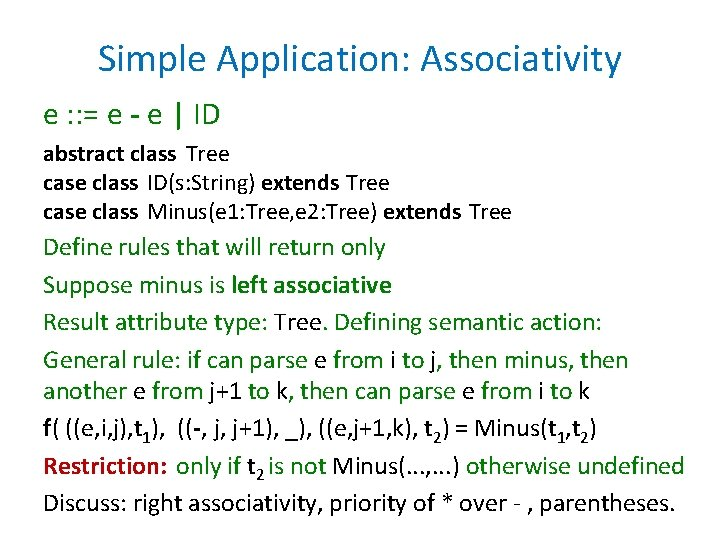Simple Application: Associativity e : : = e - e | ID abstract class Tree case class ID(s: String) extends Tree case class Minus(e 1: Tree, e 2: Tree) extends Tree Define rules that will return only Suppose minus is left associative Result attribute type: Tree. Defining semantic action: General rule: if can parse e from i to j, then minus, then another e from j+1 to k, then can parse e from i to k f( ((e, i, j), t 1), ((-, j, j+1), _), ((e, j+1, k), t 2) = Minus(t 1, t 2) Restriction: only if t 2 is not Minus(. . . , . . . ) otherwise undefined Discuss: right associativity, priority of * over - , parentheses.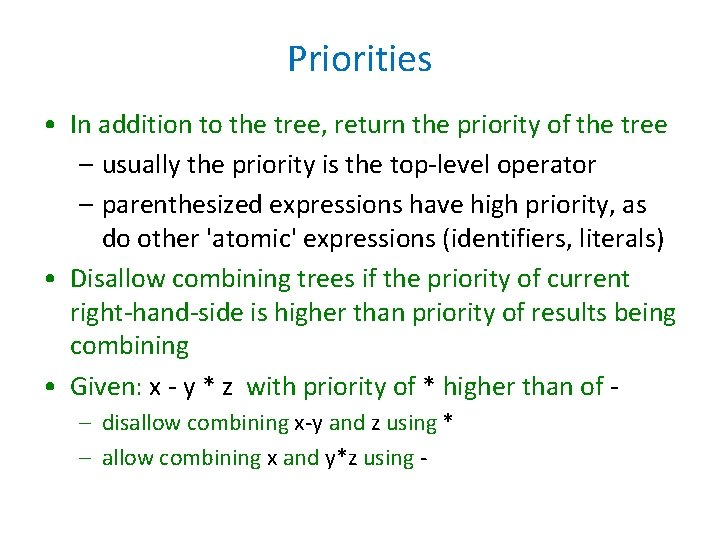Priorities • In addition to the tree, return the priority of the tree – usually the priority is the top-level operator – parenthesized expressions have high priority, as do other 'atomic' expressions (identifiers, literals) • Disallow combining trees if the priority of current right-hand-side is higher than priority of results being combining • Given: x - y * z with priority of * higher than of – disallow combining x-y and z using * – allow combining x and y*z using -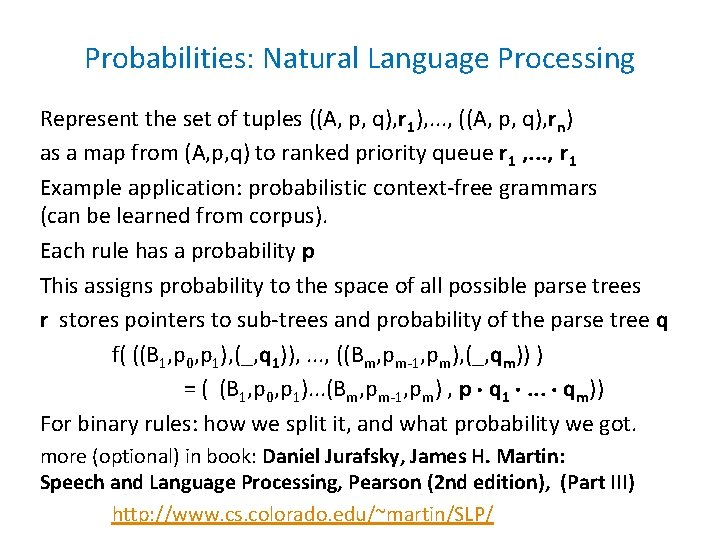Probabilities: Natural Language Processing Represent the set of tuples ((A, p, q), r 1), . . . , ((A, p, q), rn) as a map from (A, p, q) to ranked priority queue r 1 , . . . , r 1 Example application: probabilistic context-free grammars (can be learned from corpus). Each rule has a probability p This assigns probability to the space of all possible parse trees r stores pointers to sub-trees and probability of the parse tree q f( ((B 1, p 0, p 1), (_, q 1)), . . . , ((Bm, pm-1, pm), (_, qm)) ) = ( (B 1, p 0, p 1). . . (Bm, pm-1, pm) , p q 1 . . . qm)) For binary rules: how we split it, and what probability we got. more (optional) in book: Daniel Jurafsky, James H. Martin: Speech and Language Processing, Pearson (2 nd edition), (Part III) http: //www. cs. colorado. edu/~martin/SLP/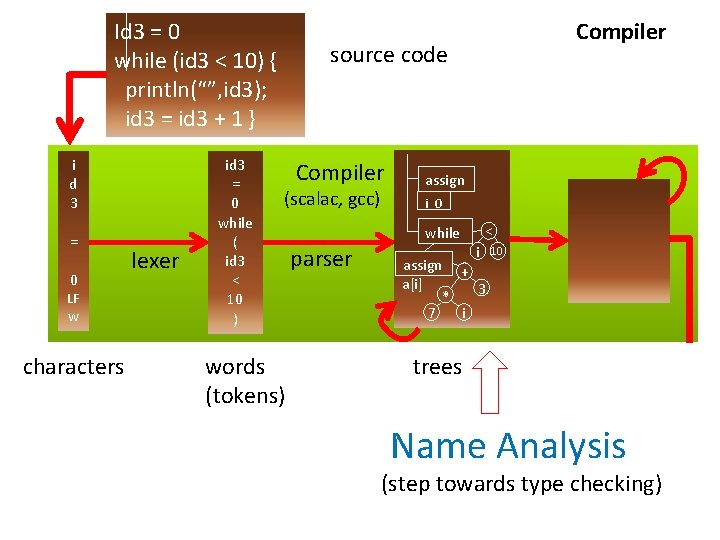Id 3 = 0 while (id 3 < 10) { println(“”, id 3); id 3 = id 3 + 1 } i d 3 = 0 LF w characters lexer id 3 = 0 while ( id 3 < 10 ) Compiler source code Compiler (scalac, gcc) words (tokens) assign i 0 while parser < i assign + a[i] 3 * 7 i 10 trees Name Analysis (step towards type checking)Name Analysis Problems Detected • a class is defined more than once: class A {. . . } class B {. . . } class A {. . . } • a variable is defined more than once: int x; int y; int x; • a class member is overloaded (forbidden in Tool, requires override keyword in Scala): class A { int x; . . . } class B extends A { int x; . . . } • a method argument is shadowed by a local variable declaration (forbidden in Java, Tool): def (x: Int) { var x : Int; . . . } • two method arguments have the same name: def (x: Int, y: Int, x: Int) {. . . } • a class name is used as a symbol (as parent class or type, for instance) but is not declared: class A extends Objekt {} • an identifier is used as a variable but is not declared: def(amount: Int ) { total = total + ammount } • the inheritance graph has a cycle: class A extends B {} class B extends C {} class C extends A To make it efficient and clean to check for such errors, we associate mapping from each identifier to the symbol that the identifier represents. • We use Map data structures to maintain this mapping (Map, what else? ) • The rules that specify how declarations are used to construct such maps are given by scoping rules of the programming language.Example: program result, symbols, scopes class Example { Scope of a variable = part of program where it is visible boolean x; Draw an arrow from occurrence of int y; each identifier to the point of its int z; declaration. int compute(int x, int y) { For each declaration of identifier, int z = 3; identify where the identifier can be return x + y + z; referred to (its scope). } public void main() { Name analysis: int res; • compute those arrows = maps, partial functions (math) x = true; = environments (PL theory) y = 10; = symbol table (implementation) z = 17; • report some simple semantic errors res = compute(z, z+1); System. out. println(res); Usually introduce symbols for things denoted by identifiers. } Symbol tables map identifiers to symbols. }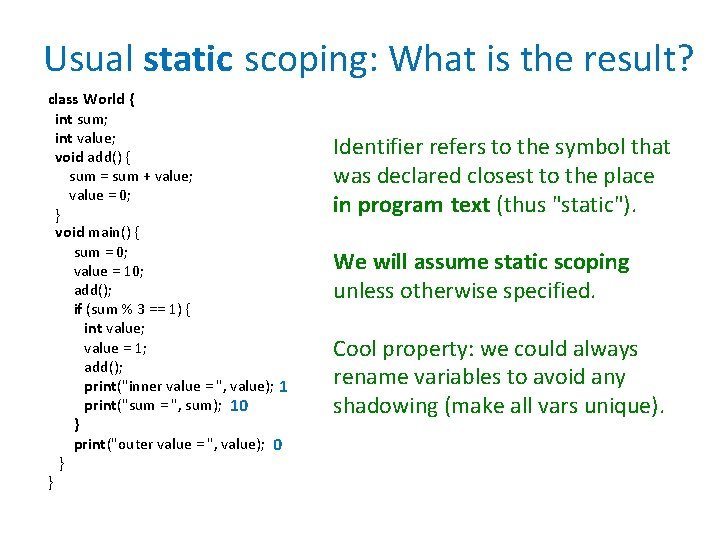Usual static scoping: What is the result? class World { int sum; int value; void add() { sum = sum + value; value = 0; } void main() { sum = 0; value = 10; add(); if (sum % 3 == 1) { int value; value = 1; add(); print("inner value = ", value); 1 print("sum = ", sum); 10 } print("outer value = ", value); 0 } } Identifier refers to the symbol that was declared closest to the place in program text (thus "static"). We will assume static scoping unless otherwise specified. Cool property: we could always rename variables to avoid any shadowing (make all vars unique).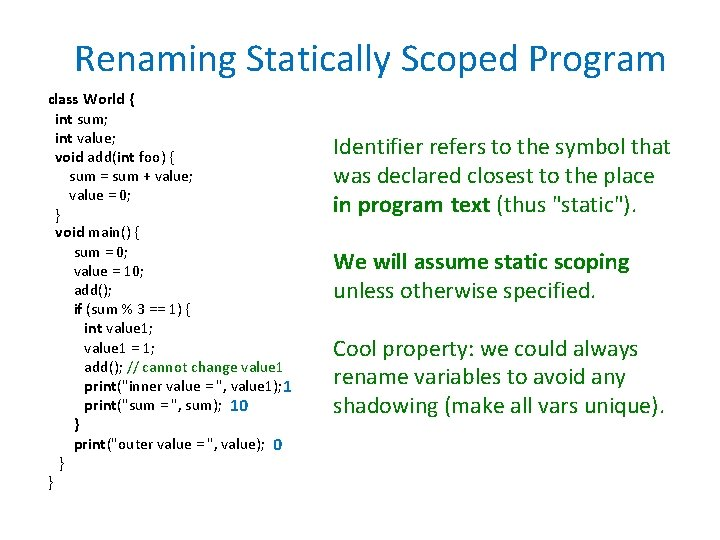Renaming Statically Scoped Program class World { int sum; int value; void add(int foo) { sum = sum + value; value = 0; } void main() { sum = 0; value = 10; add(); if (sum % 3 == 1) { int value 1; value 1 = 1; add(); // cannot change value 1 print("inner value = ", value 1); 1 print("sum = ", sum); 10 } print("outer value = ", value); 0 } } Identifier refers to the symbol that was declared closest to the place in program text (thus "static"). We will assume static scoping unless otherwise specified. Cool property: we could always rename variables to avoid any shadowing (make all vars unique).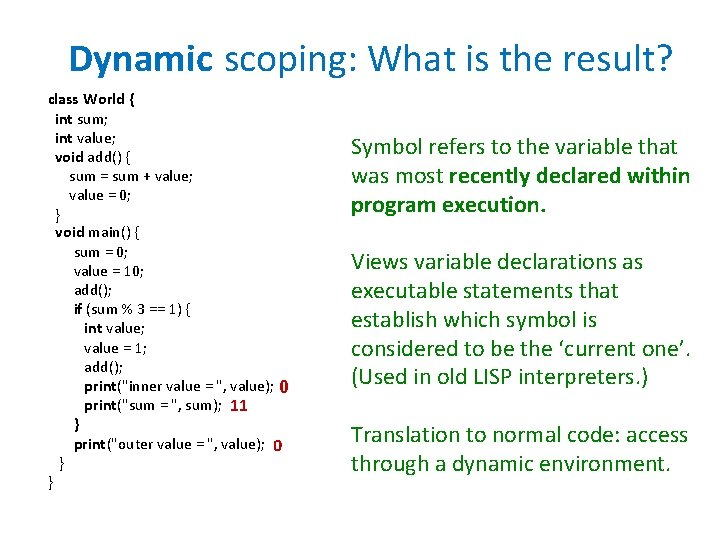Dynamic scoping: What is the result? class World { int sum; int value; void add() { sum = sum + value; value = 0; } void main() { sum = 0; value = 10; add(); if (sum % 3 == 1) { int value; value = 1; add(); print("inner value = ", value); 0 print("sum = ", sum); 11 } print("outer value = ", value); 0 } } Symbol refers to the variable that was most recently declared within program execution. Views variable declarations as executable statements that establish which symbol is considered to be the ‘current one’. (Used in old LISP interpreters. ) Translation to normal code: access through a dynamic environment.Dynamic scoping translated using global map, working like stack class World { int sum; int value; void add() { sum = sum + value; value = 0; } void main() { sum = 0; value = 10; add(); if (sum % 3 == 1) { int value; value = 1; add(); print("inner value = ", value); 0 print("sum = ", sum); 11 } print("outer value = ", value); 0 } } class World { push. New. Declaration('sum); push. New. Declaration('value); void add(int foo) { update('sum, lookup('sum) + lookup('value)); update('value, 0); } void main() { update('sum, 0); update('value, 10); add(); if (lookup('sum) % 3 == 1) { push. New. Declaration('value); update('value, 1); add(); print("inner value = ", lookup('value)); print("sum = ", lookup('sum)); pop. Declaration('value) } print("outer value = ", lookup('value)); } }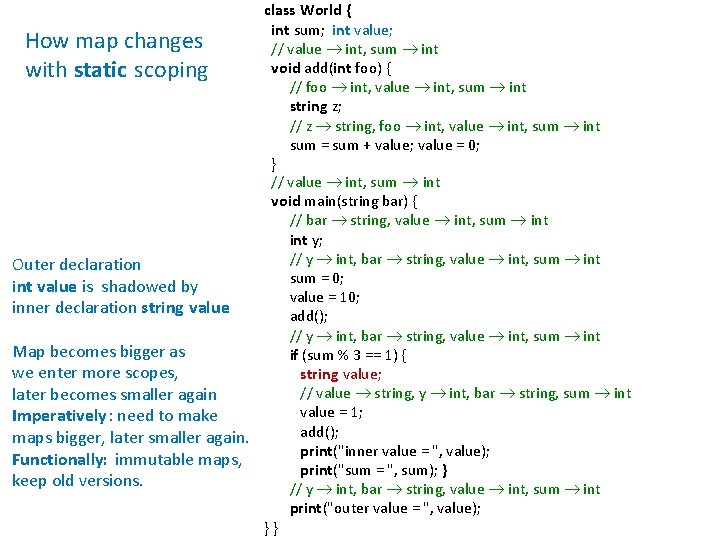class World { int sum; int value; How map changes // value int, sum int void add(int foo) { with static scoping // foo int, value int, sum int string z; // z string, foo int, value int, sum int sum = sum + value; value = 0; } // value int, sum int void main(string bar) { // bar string, value int, sum int y; // y int, bar string, value int, sum int Outer declaration sum = 0; int value is shadowed by value = 10; inner declaration string value add(); // y int, bar string, value int, sum int Map becomes bigger as if (sum % 3 == 1) { we enter more scopes, string value; // value string, y int, bar string, sum int later becomes smaller again value = 1; Imperatively: need to make add(); maps bigger, later smaller again. print("inner value = ", value); Functionally: immutable maps, print("sum = ", sum); } keep old versions. // y int, bar string, value int, sum int print("outer value = ", value); }}Abstract Trees of Simple Language with Arbitrarily Nested Blocks program : : = class World { var. Decl* method* } method : : = var. Decl ( var. Decl* ) { thing* return expr } var. Decl : : = type ID type : : = int | boolean | void thing : : = var. Decl | stmt : : = expr | if | while | block if : : = if (expr) stmt else stmt while : : = while expr stmt block : : = { thing* } expr : : = ID | expr + expr | expr <= expr| assign | call | cond. Expr assign : : = ID = expr cond. Expr : : = expr ? expr : expr call : : = ID ( expr* )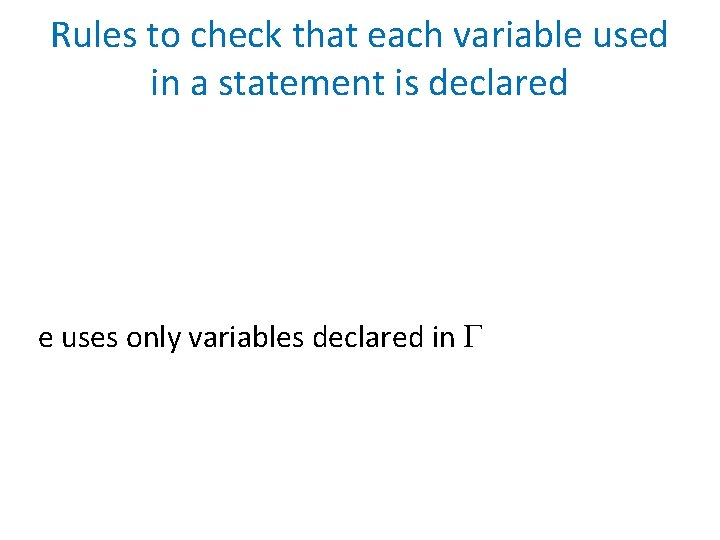Rules to check that each variable used in a statement is declared e uses only variables declared in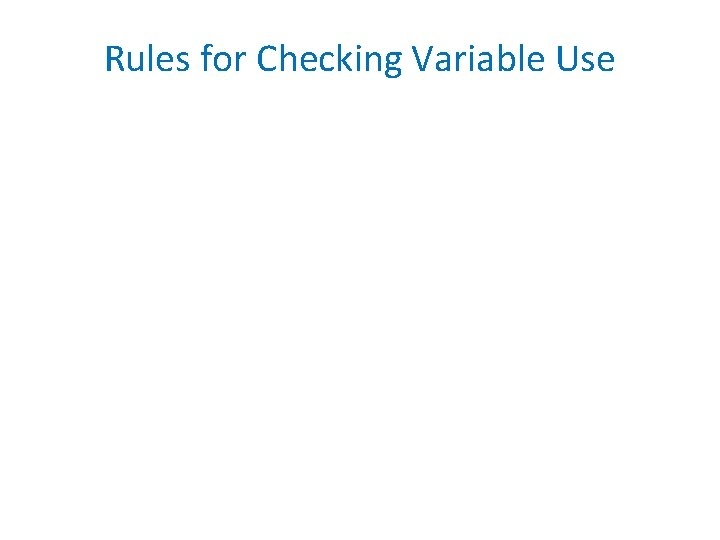Rules for Checking Variable UseLocal Block Declarations Change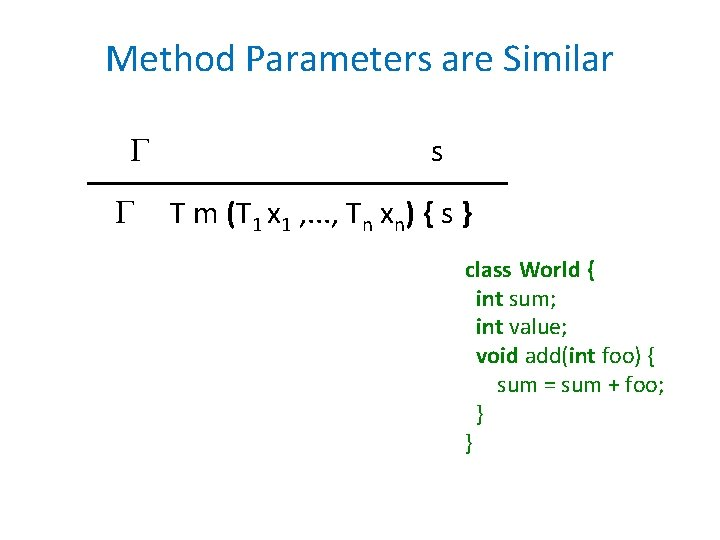Method Parameters are Similar s T m (T 1 x 1 , . . . , Tn xn) { s } class World { int sum; int value; void add(int foo) { sum = sum + foo; } }